﻿ 船舶动力定位自适应滤波器设计
 舰船科学技术2017, Vol. 39Issue (10): 79-83PDF

Adaptive filter design for ship dynamic positioning
FAN Ji-sheng, YUAN Wei, LI Wen-juan, JIN Yue
Jiangsu University of Science and Technology, Zhenjiang 212003, China
Abstract: Although the traditional notch filter can filter out the 1st-order disturbances,it will also make the signal has a serious phase lag,whose performance in the time domain is the time delay.Kalman filter can solve the problem well,but it depends on the ship model very much.This paper aims at the defects of the traditional notch filter using a integral compensation notch filter,which can improve the traditional filter.A simulation experiment based on a tugboat shows this filter not only can well remove high frequency wave,but also can maintain good phase characteristics.At the same time,the Recursive least squares is used to estimate the dominant wave frequency,which makes the filter have better adaptability.
Key words: dynamic positioning     phase lag     notch filter     adaptability
0 引 言

1 海浪的高频模型

 $h(s) = \frac{{{K_\omega }s}}{{{s^2} + 2\varsigma {\omega _0}s + \omega _0^2}},$ (1)

 $\ddot y(t) + 2\varsigma {\omega _0}\dot y(t) + \omega _0^2y(t) = {K_\omega }\dot \omega (t),$ (2)

 $\left\{ \begin{array}{l}{{\dot X}_H} = {A_H}{X_H} + {E_H}{w_H},\\[4pt]{Y_H} = {C_H}{X_H}\text {。}\end{array} \right.$ (3)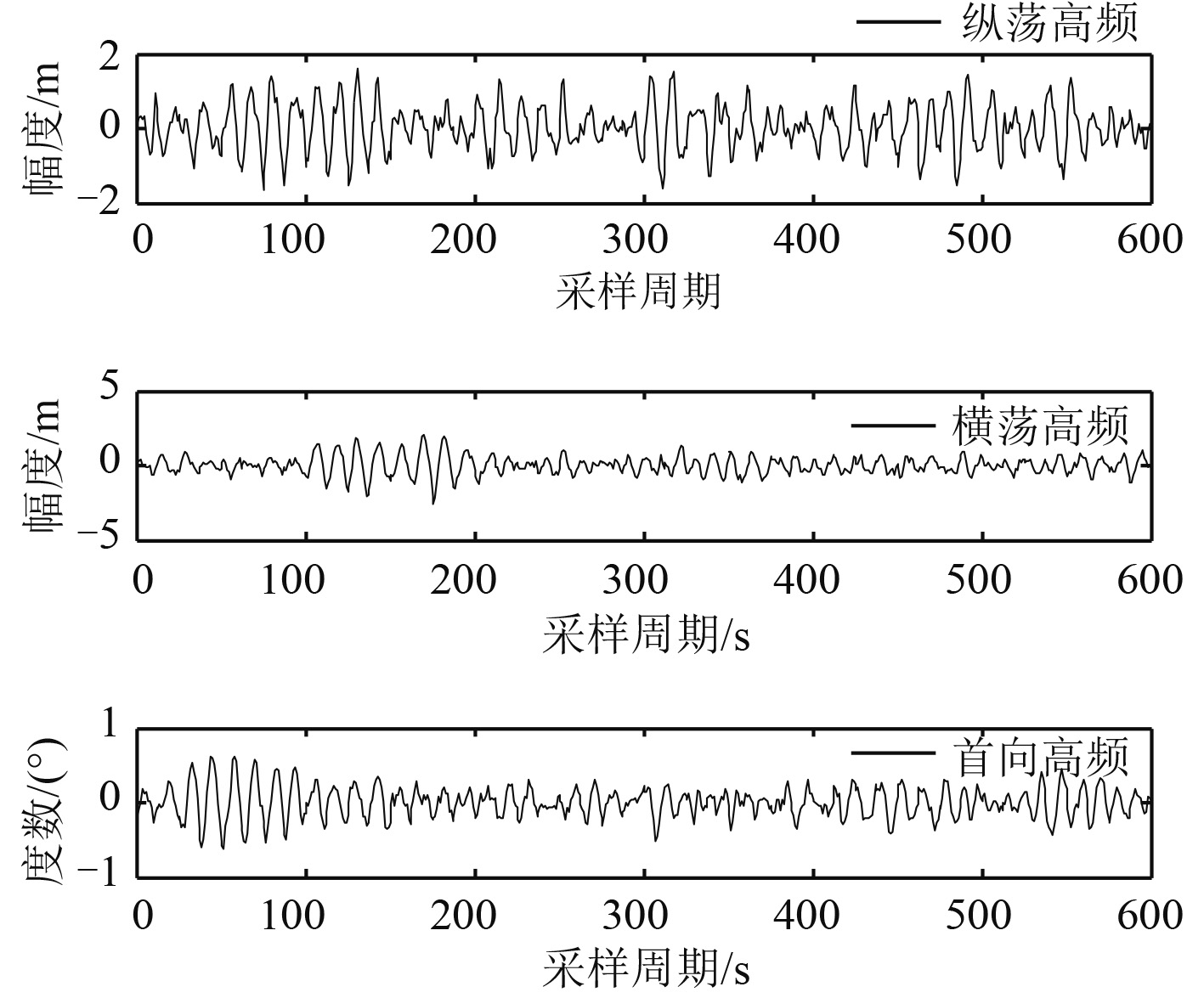图 1 高频波浪的输出 Fig. 1 Output of high frequency waves
2 改进的滤波器系统框图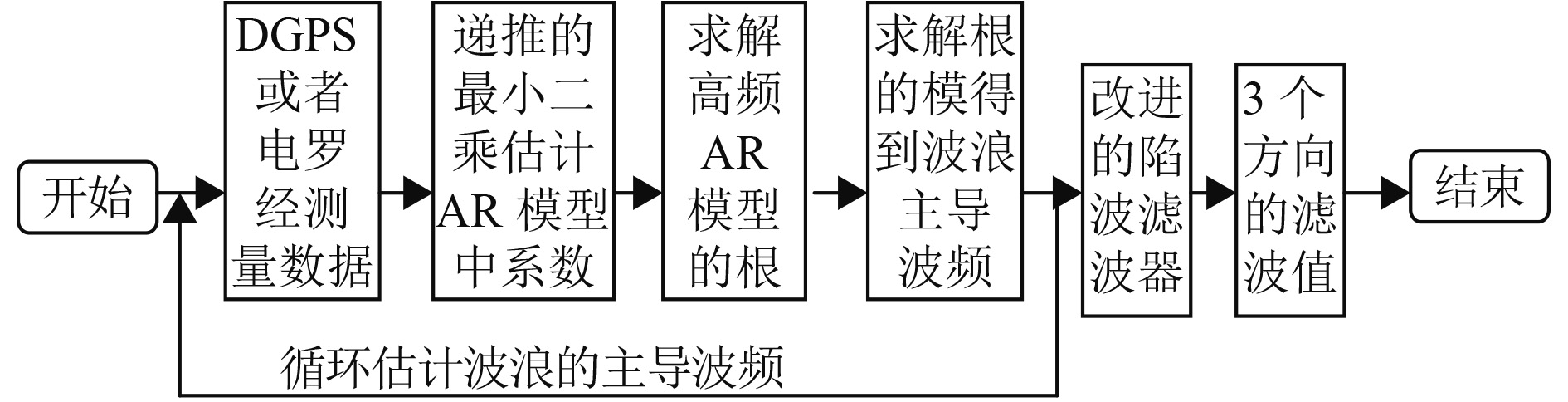图 2 滤波流程图 Fig. 2 Filtering flow chart
3 递推的最小二乘波频估计

AR 模型的形式如下：

 $A({z^{ - 1}})y(k) = e(k)\text{。}$ (4)

 $\psi (s) = {\psi _L}(s) + {\psi _H}(s),$ (5)

 ${\psi _H}(s) = \frac{{{T_f}s}}{{1 + {T_f}s}}\psi (s)\text{。}$ (6)

 ${\xi _H}(s)\! =\!\! \frac{1}{s}{\psi _H}(s) \!= \!\!\frac{{{T_f}}}{{1 + {T_f}s}}\psi (s) \!=\!\! \frac{{{K_w}}}{{{s^2} + 2\zeta ws + {w^2}}}\omega (s)\text{。}\!\!\!$ (7)

 $\begin{array}{l}A({z^{ - 1}}) = 1 + {a_1}{z^{ - 1}} + {a_2}{z^{ - 2}} + {a_3}{z^{ - 3}} = 0,\\[5pt]e(k) = {K_w}w(k)\text{。}\end{array}$ (8)

a1a2a3 的估计通过带遗忘因子的递推最小二乘估计得到的：

 $\begin{array}{l}\hat \theta (k) = \hat \theta (k - 1) + K(k)[y(k) - {\phi ^{\rm T}}(k)\hat \theta (k - 1)],\\[5pt]K(k) = \displaystyle\frac{{P(k - 1)\phi (k)}}{{\lambda + {\phi ^T}(k)P(k - 1)\phi (k)}},\\[8pt]P(k) = \frac{1}{\lambda }[I - K(k){\phi ^{\rm T}}(k)]P(k - 1)\text{。}\end{array}$ (9)表 1 高频波浪数据 Tab.1 Data of high frequency wave

RLS 仿真估计波浪频率的结果如图 3 所示。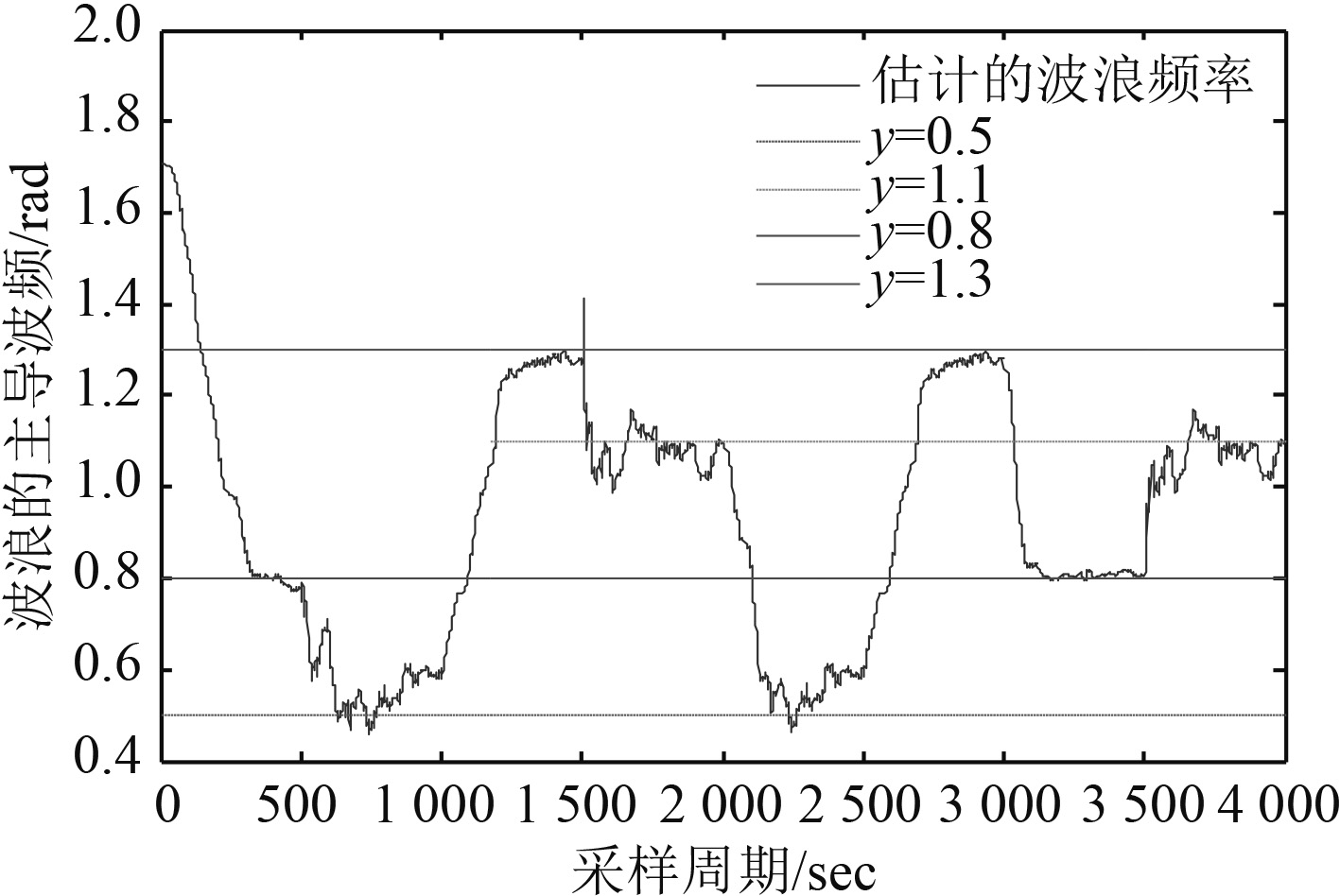图 3 递推的最小二乘波频估计 Fig. 3 Recursive least square wave frequency estimation

4 改进的陷波滤波器

 $H(s) = \frac{{{s^2} + {w^2}}}{{{s^2} + kws + {w^2}}} = 1 - \frac{{kws}}{{{s^2} + kws + {w^2}}} = 1 - Q,$ (10)

Q 转化为状态空间的形式：

 $\begin{array}{l}\mathop {{x_1}}\limits^ \bullet = w{x_2},\\\mathop {{x_2}}\limits^ \bullet = - w{x_1} + kw(u - {x_2})\text{。}\end{array}$ (11)

 $\begin{array}{l}\mathop {{x_1}}\limits^ \bullet = w{x_2},\\\mathop {{x_2}}\limits^ \bullet = - w{x_1} + kw(u - {x_2} - {x_3}),\\\mathop {{x_3}}\limits^ \bullet = aw(u - {x_2} - {x_3})\text{。}\end{array}$ (12)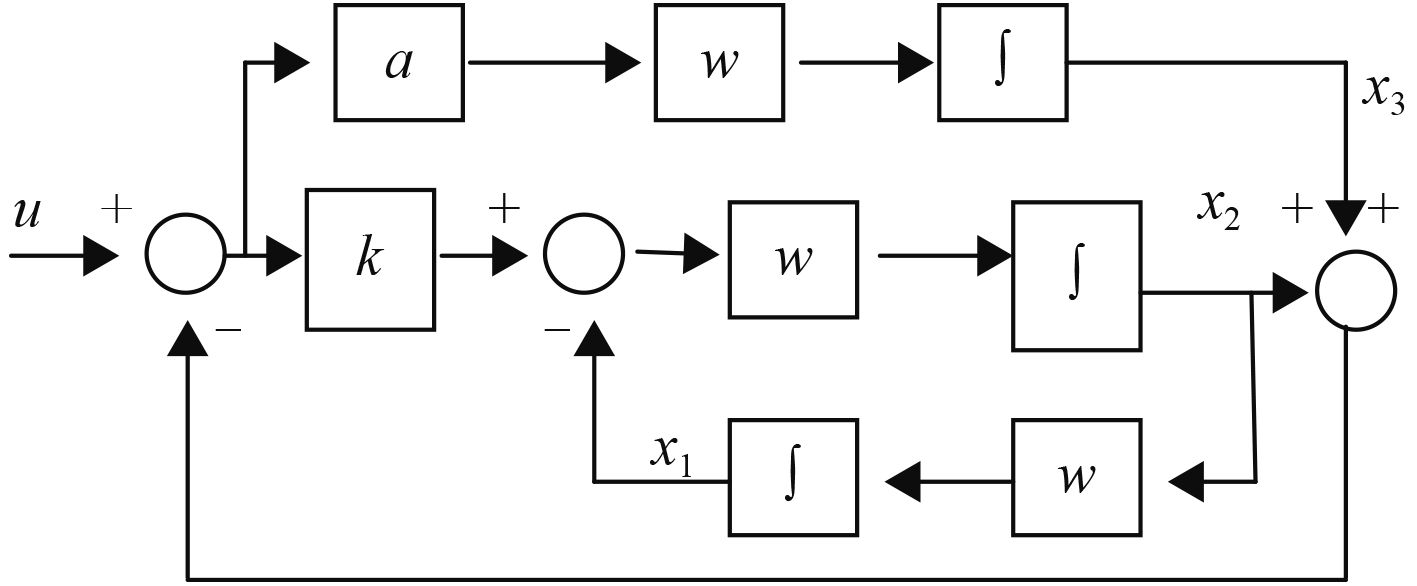图 4 改进的滤波框图 Fig. 4 Improved filter block diagram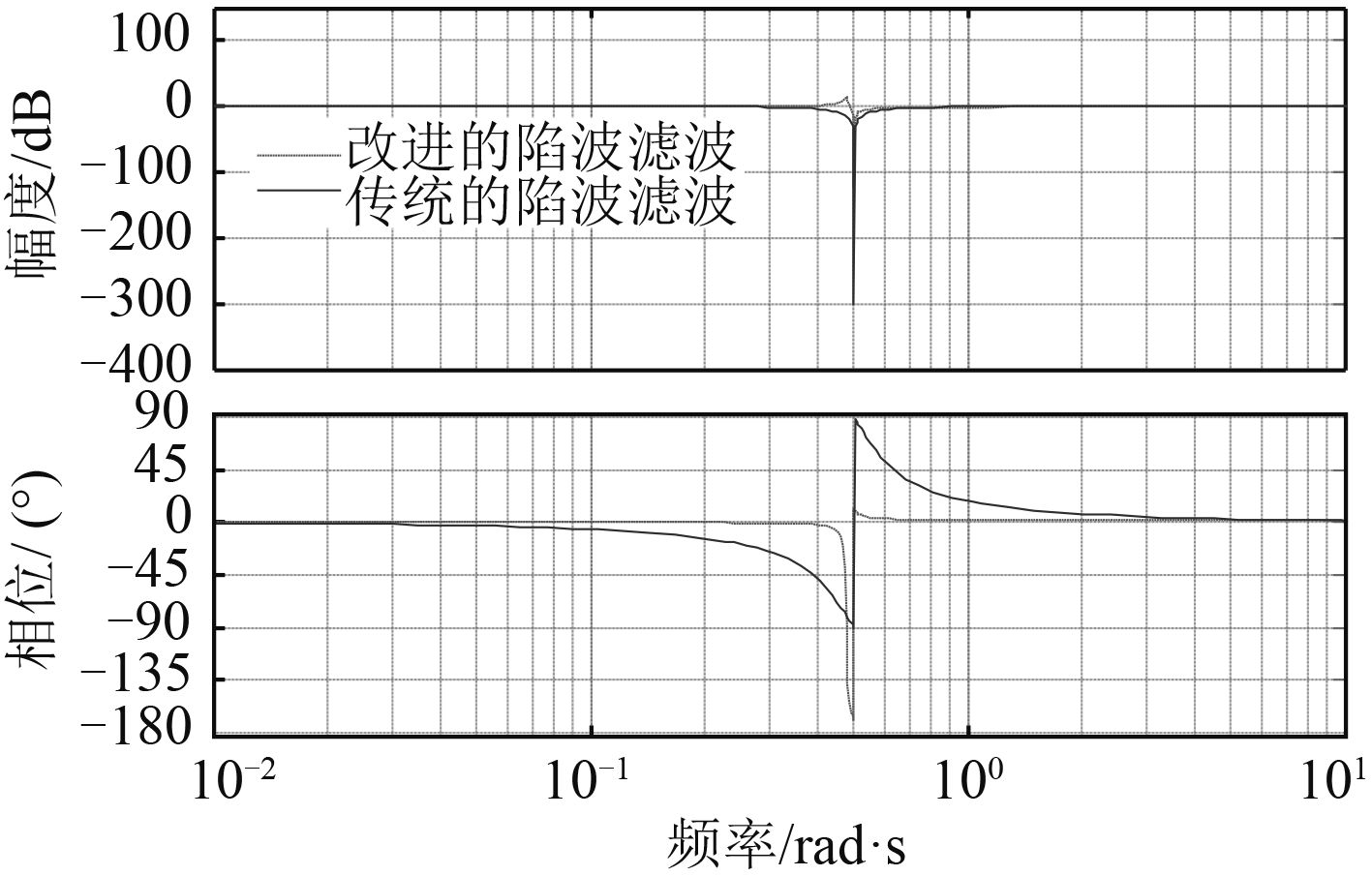图 5 改进滤波器的伯德图 Fig. 5 Improved filter Bode diagram

5 仿真实验表 2 仿真船舶的主要参数 Tab.2 Main parameters of simulation ship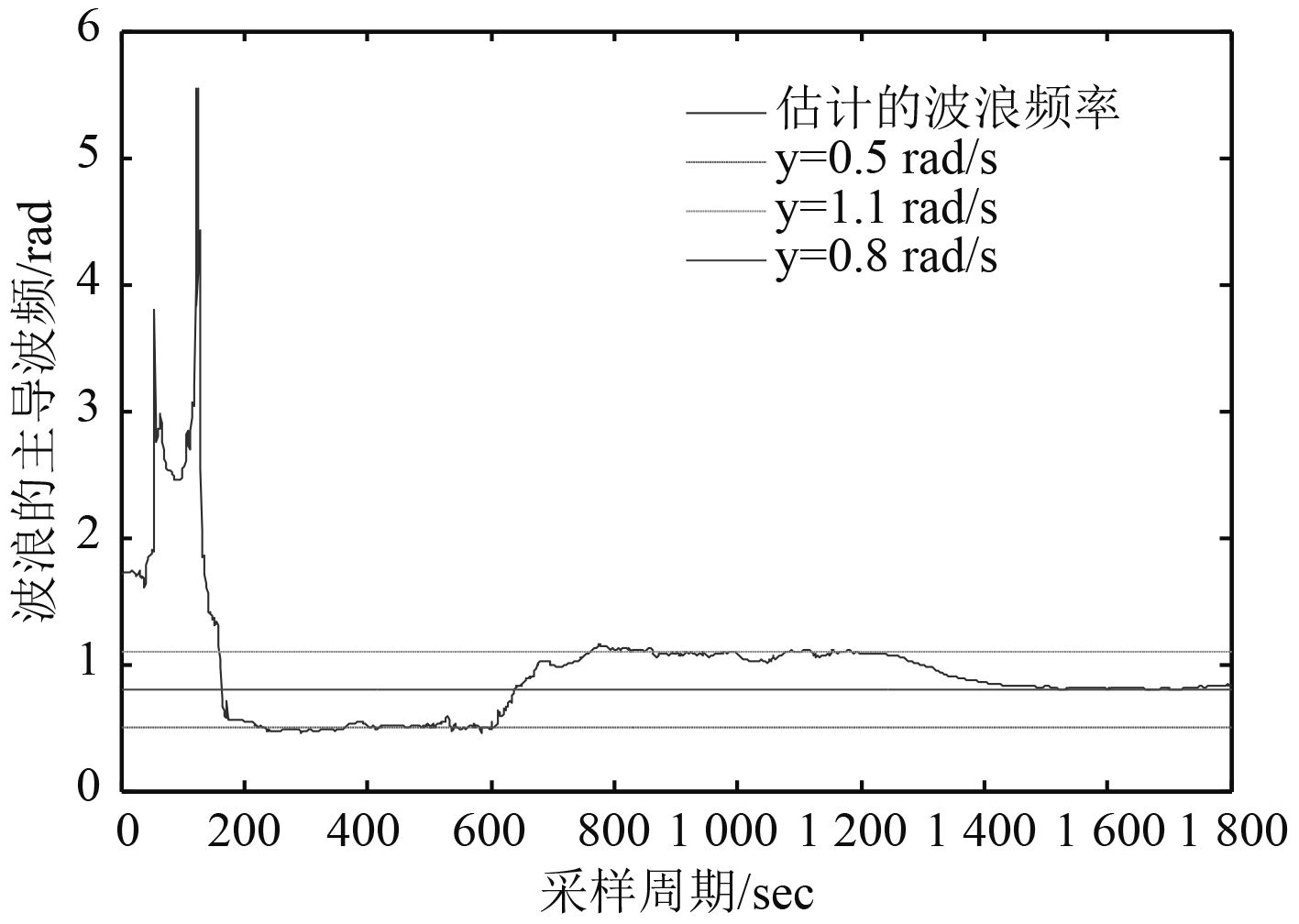图 6 波频估计 Fig. 6 Wave frequency estimation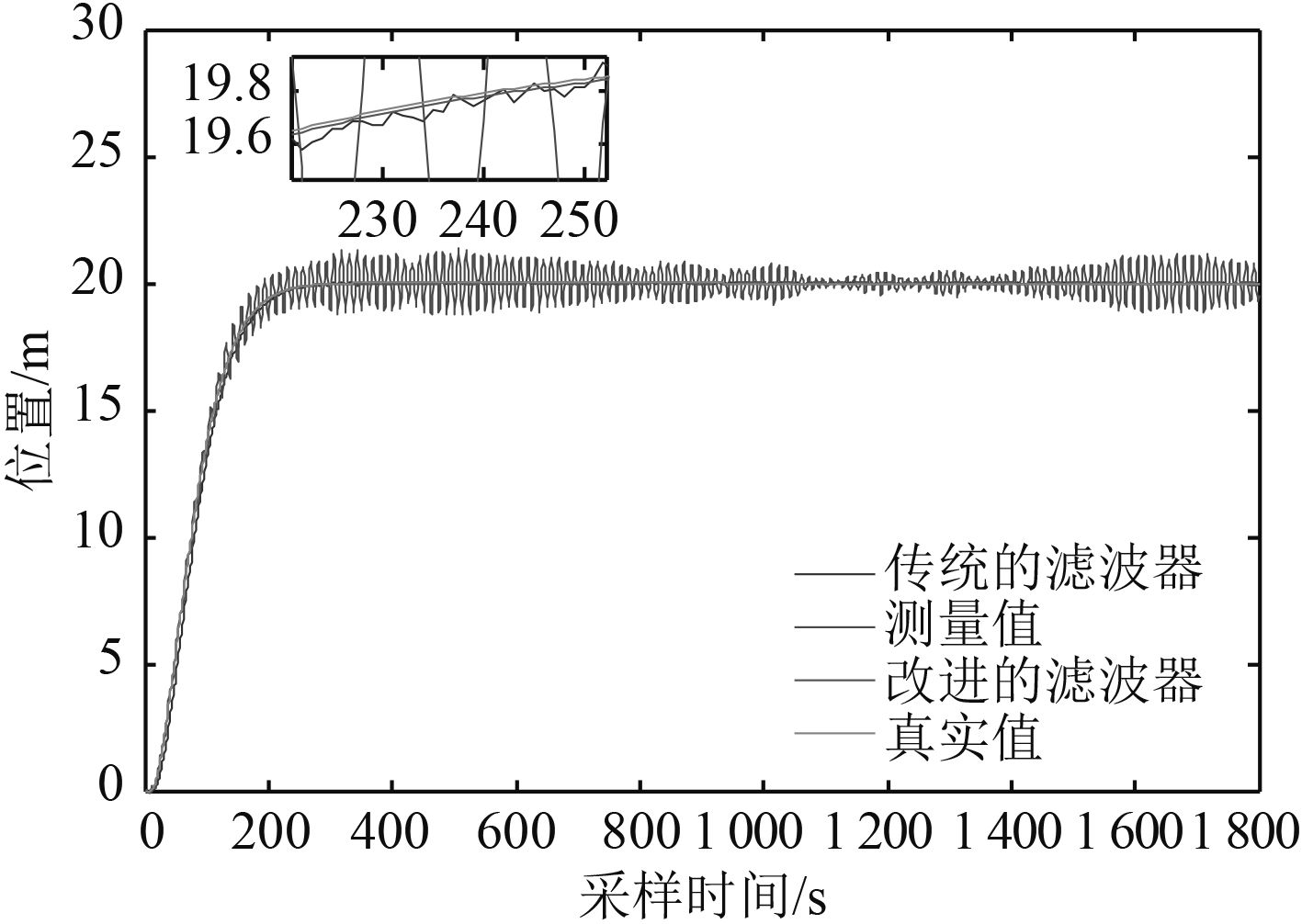图 7 船舶纵荡的对比 Fig. 7 Comparision of the direction of surge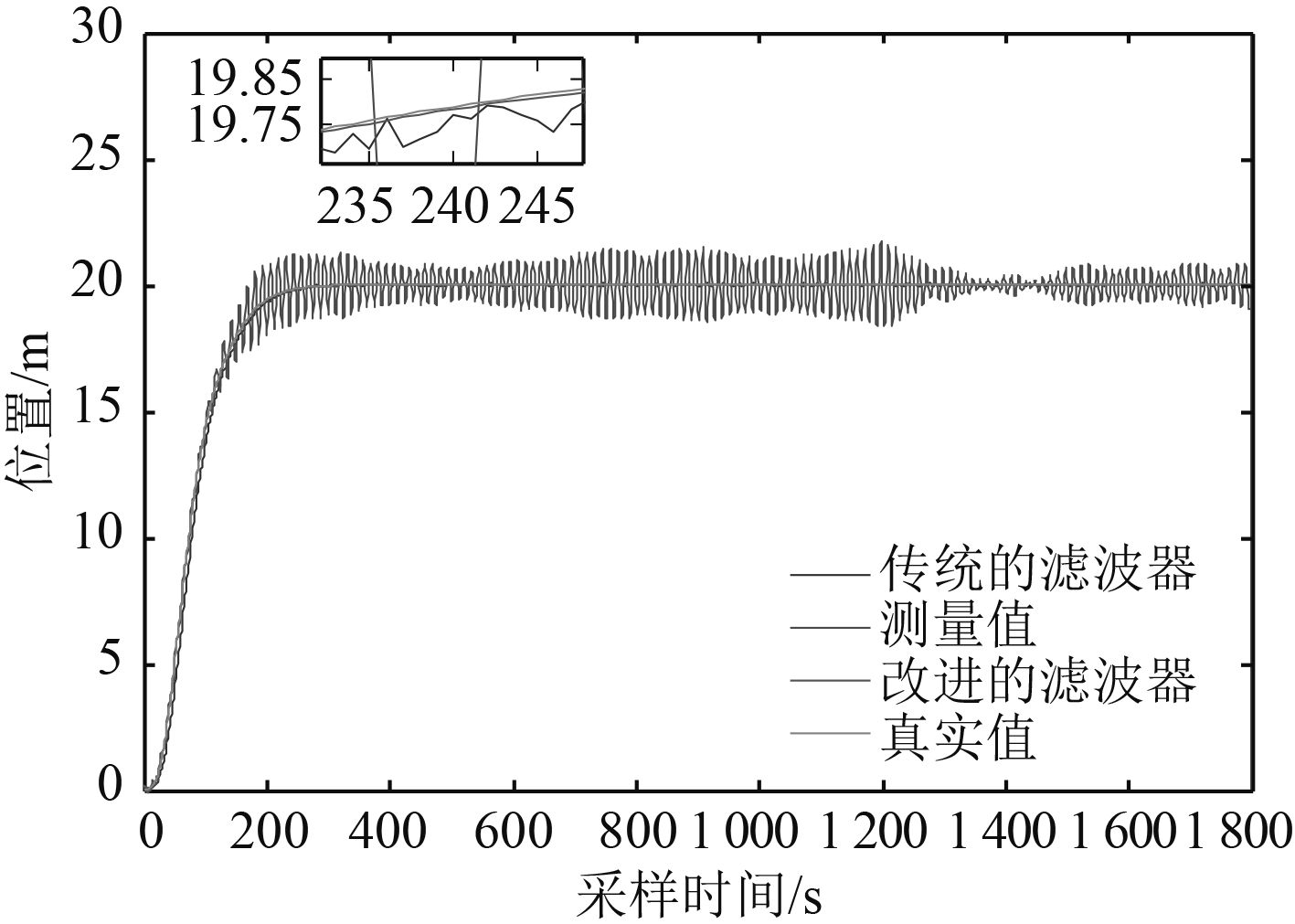图 8 船舶横荡对比 Fig. 8 Comparision of the direction of sway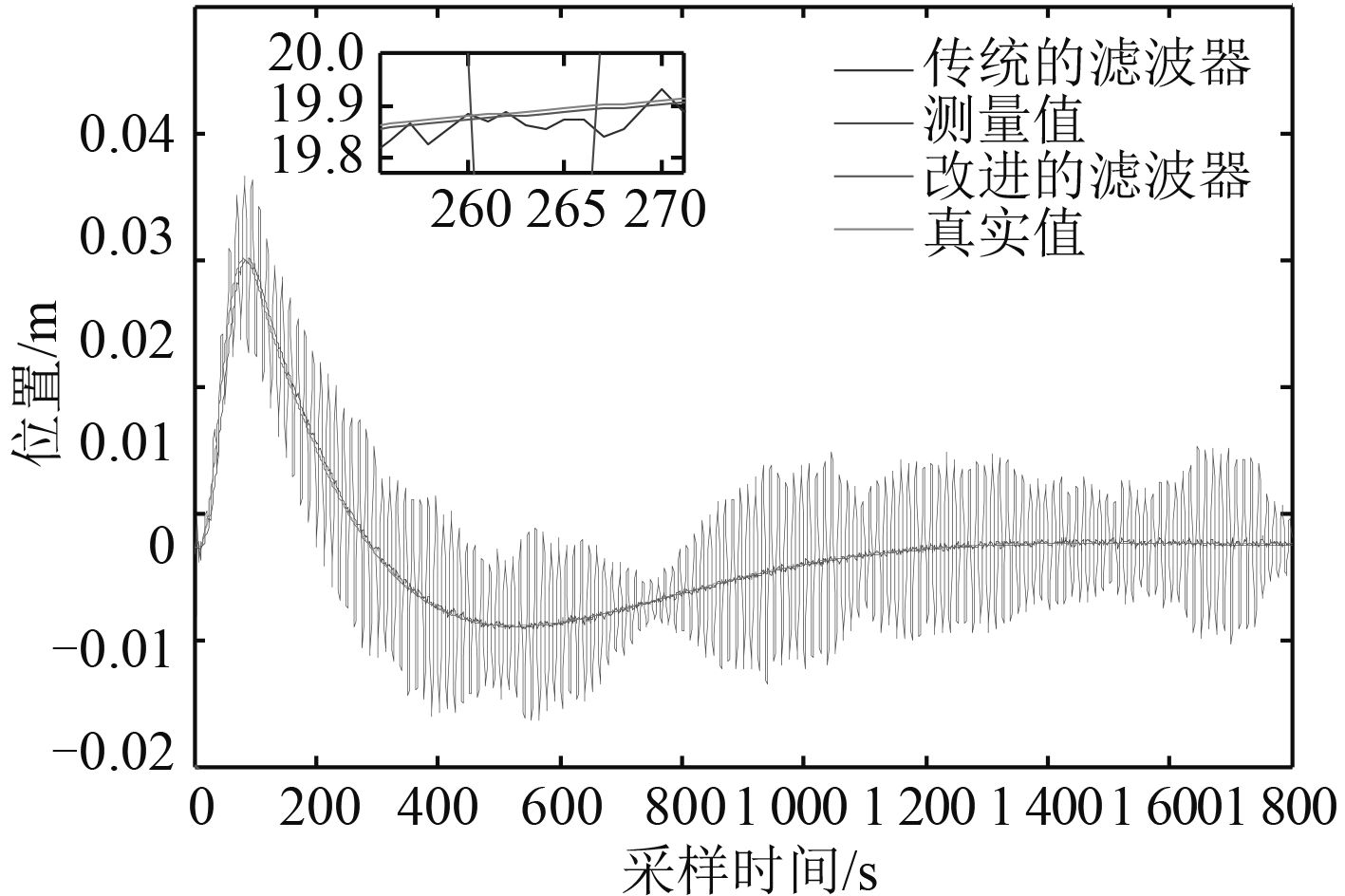图 9 船舶首向对比 Fig. 9 Comparision of the direction of yaw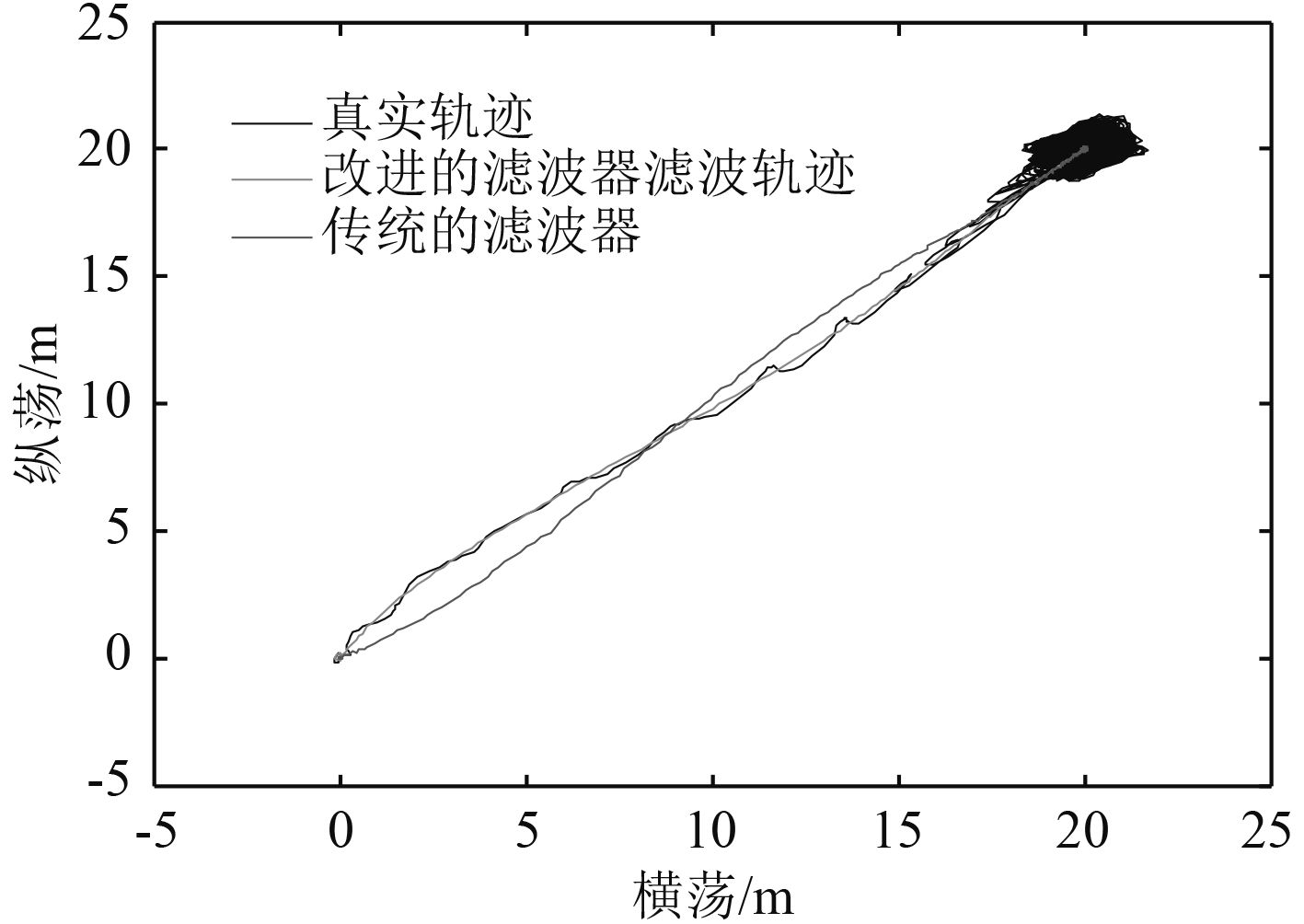图 10 船舶行走轨迹对比 Fig. 10 Comparison of ship's walking trajectory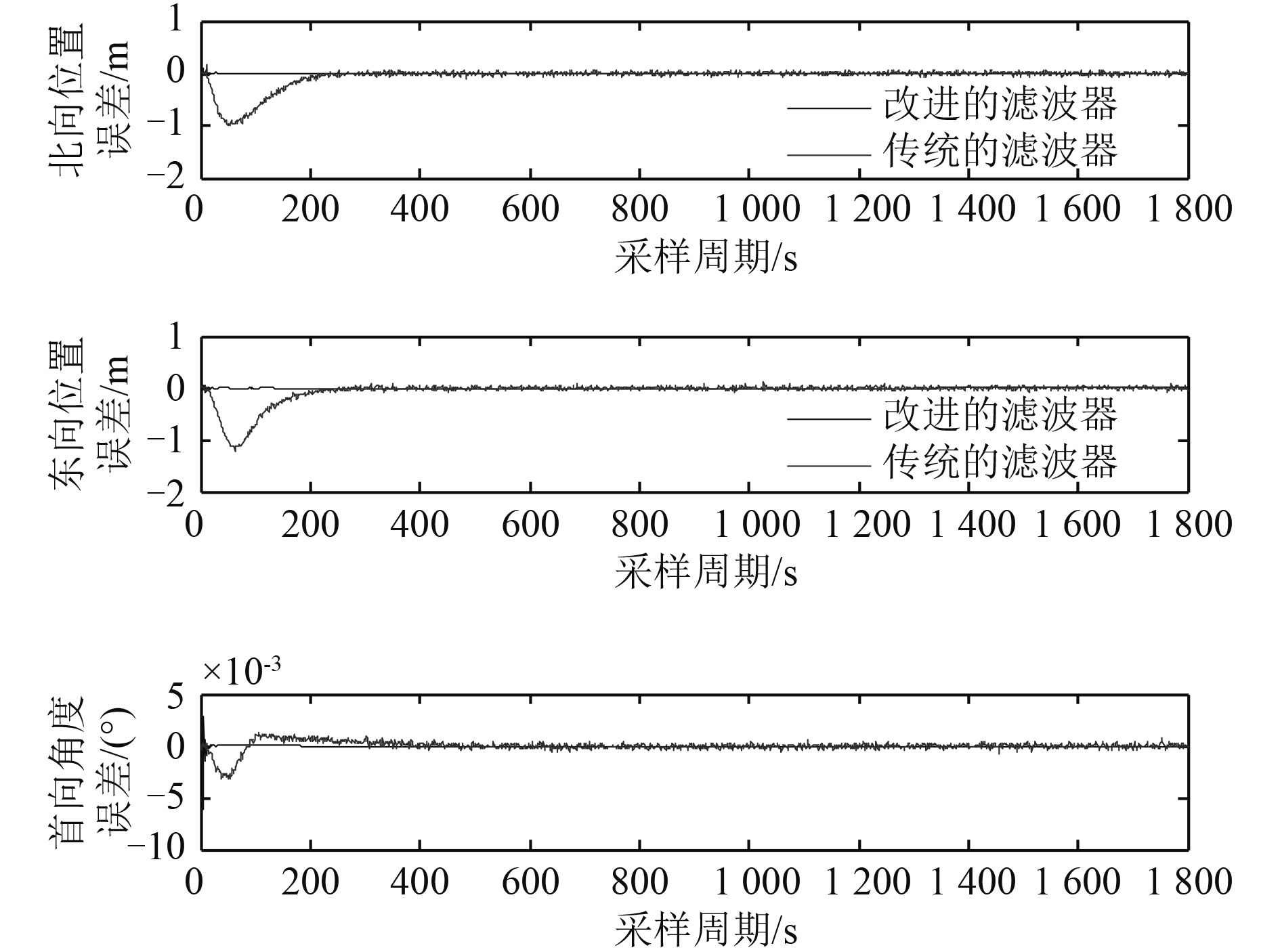图 11 误差对比 Fig. 11 Error contrast

6 结 语

  边信黔, 付明玉, 王元慧. 船舶动力定位[M]. 北京: 科学出版社, 2011.  FOSSENT I. Kalman filtering for positioning and heading control of ships and offshore rigs[J]. IEEE Control Systems, 2009, 29 (6): 32–46. DOI: 10.1109/MCS.2009.934408  FOSSEN T I. Guidance and control of ocean vehicles[M]. Chichester, UK: John Wiley and Sons, 1994.  张贤达. 现代信号处理[M]. 北京: 清华大学出版社, 1995. ZHANG Xian-da. Modern signal processing[M]. Beijing: Tsinghua University Press, 1995.  LOUEIPOURM. Wave filtering and state estimation in dynamic positioning of marine vessels using position measurement[J]. IEEE Transactions on Instrumentation and Measurement, 2015, 64 (12): 3253–3261. DOI: 10.1109/TIM.2015.2459551  齐国鹏, 俞孟蕻, 袁伟. 动力定位系统自适应滤波技术[J]. 舰船科学技术, 2013, 35 (10): 96–100. QIGuo-peng, YUMeng-hong, YUANWei. Research on adaptive filtering techniques in dynamic positioning systems[J]. Ship Science and Technology, 2013, 35 (10): 96–100. DOI: 10.3404/j.issn.1672-7649.2013.10.022  FOSSEN T I. Marine control systems-guidance, navigation, and control of ships, rigs and underwater vehicles[M]. Norway: Marine Cybernetics, 2002.  TANNURI E A, BRAVIN T T, PESCE C P. Dynamic positioning systems: comparison between wave filtering algorithms and their influence on performance[C]//Proceedings of ASME 2003 22nd International Conference on Offshore Mechanics and Arctic Engineering. Cancun, Mexico: ASME, 2003: 109–117.  FAŸ H. Dynamic positioning systems principles, design and applications[M]. Paris: Editions Technip, 1990.